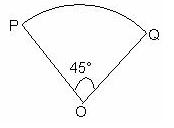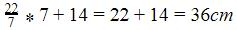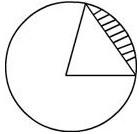Courses

# Test: Area And Perimeter Of A Circle

## 25 Questions MCQ Test Mathematics (Maths) Class 10 | Test: Area And Perimeter Of A Circle

Description
This mock test of Test: Area And Perimeter Of A Circle for Class 10 helps you for every Class 10 entrance exam. This contains 25 Multiple Choice Questions for Class 10 Test: Area And Perimeter Of A Circle (mcq) to study with solutions a complete question bank. The solved questions answers in this Test: Area And Perimeter Of A Circle quiz give you a good mix of easy questions and tough questions. Class 10 students definitely take this Test: Area And Perimeter Of A Circle exercise for a better result in the exam. You can find other Test: Area And Perimeter Of A Circle extra questions, long questions & short questions for Class 10 on EduRev as well by searching above.
QUESTION: 1

### A wire is bent in the form of a circle of radius 28 cm. It is bent to form a square. The length of the side of the square will be​

Solution:

Circumference of a circle = 2πr
= 2*22/7*28
= 2*22*4
= 176
now, perimeter of square = circumference of a circle = 176
Therefore, side of square = perimeter/4
= 176/4
= 44 answer.

QUESTION: 2

### The radius of a circle if its perimeter and area are numerically equal is​

Solution:

Perimeter of circle = 2πr
Area of circle= πr2
Perimeter = Area
2πr= πr2
2= πr2/r
πr gets cancelled
So r = 2 units

QUESTION: 3

### The diameter of a cycle wheel is 28 cm. The number of revolutions it makes in moving 13.2 km is​

Solution:
QUESTION: 4

Find the circumference of the circle, whose area is 144π cm2

Solution:

The area should be 144π,
,Area of the circle= πr2
144π = πr2
r = 12cm
Circumference=2πr = 24πcm

QUESTION: 5

The area of a circular pizza is 616 cm2. Its diameter is​

Solution:
QUESTION: 6

The diameter of a circle whose area is equal to the sum of the areas of the two circles of radii 40 cm and 9 cm is:​

Solution:

Area of the circle = sum of area of two circlesQUESTION: 7

The area of a circle with diameter 6 m exceeds the combined areas of circles with diameters 4m and 2 m by​

Solution:
QUESTION: 8

The area of a circular plot is 9856 sq. m. The cost of fencing the plot at the rate of Rs. 6 per meter will be​

Solution:
QUESTION: 9

The perimeter (in cm) of a square circumscribing a circle of radius a cm, is​

Solution:

Let ABCD is a square circumscribing a circle of radius a cm.
The side of square ABCD = Diameter of circle
⇒AB=2a
Therefore, perimeter of square AB=4×AB=4×2a=8 cm

QUESTION: 10

If the circumference of a circle increases from 2 to 4 then its area is

Solution:
QUESTION: 11

A sector of a circle of radius 10.5 cm subtends an angle of 45° at the centre of the circle as in adjoining figure. Find the perimeter of sector.Solution:
QUESTION: 12

If the side of an equilateral triangle and the radius of a circle are equal, find the ratio of their areas.

Solution:
QUESTION: 13

The radii of two circles are 19 cm and 9 cm respectively. The radius of the circle which has its circumference equal to the sum of the circumferences of the two circles is:​

Solution:
QUESTION: 14

The sum of the diameters of two circles is 280 cm and the difference of their circumferences is 88 cm. Then the larger of the two radii is​

Solution:
QUESTION: 15

If the perimeter and area of a circle are numerically equal, then the radius of the circle is​

Solution:
QUESTION: 16

If the sum of the areas of two circles with radii R1 and R2 is equal to the area of a circle of radius R, then

Solution:

Area of circle with radius R= πR12
Area of circle with radius R= πR22
Area of circle with radius R = πR2
Area of circle with radius R1+Area of circle with radius R2 =Area of circle with radius R
πR1+ πR22=πR2
R12+R22=R2

QUESTION: 17

If the difference between the circumference and the radius of a circle is 37 cm, then using π = 22/7, the circumference (in cm) of the circle is:​

Solution:
QUESTION: 18

The length of the line that marks out a circle and its region is the

Solution:
QUESTION: 19

If C is the circumference of a circle of radius r, then perimeter of one of the quadrants will be​

Solution:
QUESTION: 20

If the diameter of semicircular protractor is 14 cm, then its perimeter is:​

Solution:

Diameter=14, Radius=d/2=14/2=7cm
Perimeter of the semi- circle= Boundary of the semi-circle =Circumference of the semi-circle +Diameter= πr+D=QUESTION: 21

The diameter of a circle whose area is equal to the sum of the areas of the two circles of radii 24 cm and 7 cm is​

Solution:
QUESTION: 22

If the perimeter of a sector of a circle of radius 5.2 cm. is 16.4 cm. What multiple of the radius is the area of the sector?​

Solution:

We have perimeter=16.4cm
2r+arc=16.4
Arc=16.4-10.4=6cm
Area = ½*r*Arc=½*r*6=3rd which is three times r so B is the answer.

QUESTION: 23

A garden roller has a circumference of 4 m. The number of revolutions it makes in moving 40 metres are:

Solution:

We have circumference which is equal to the boundary of the circle. So we have one revolution equal to circumference of the circle which is equal to 4
Distance covered by the roller=circumference of the roller*number of revolutions
⇒ number of revolutions = distance covered by the roller/circumference of the roller
= 40/4 = 10

QUESTION: 24

The shaded part of the circle in the given figure represents aSolution:
QUESTION: 25

The ratio of radii of two circles is in the ratio of 1:5. Calculate the ratio of their perimeters.​

Solution: